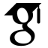Titel: End space theory for directed and undirected graphs Sprache: Englisch Autor*in: Melcher, Ruben Schlagwörter: graph theory; infinite graph theory; ends of graphs; ends of digraphs; end space theory; topological graph theory Erscheinungsdatum: 2021 Tag der mündlichen Prüfung: 2021-07-13 Zusammenfassung: This dissertation aims to make a step towards a deeper understanding of ends of infinite graphs. This first chapter serves the purpose of giving a concise introduction and an overview of the results of this dissertation. Ends of graphs are one of the most important concepts in modern infinite graph theory. They can be thought of as points at infinity to which the rays of an infinite graph converge. Formally, an end of an (undirected) graph G is an equivalence class of its rays where two rays are equivalent if for every finite vertex set X they have a tail in the same component of G-X. For example, infinite complete graphs or grids have one end while the binary tree has continuum many ends, one for every rooted ray . One typical use of ends is to extend finite to infinite theorems of graphs, in that graphs together with their ends naturally form topological spaces; in these spaces, topological arcs and circles take the role of paths and cycles, respectively. However, their use goes far beyond this.Only recently the infinite cycle space theory which ends make possible uncovered a deep connection between combinatorics and a long-standing problem in topology, see . Within combinatorics, ends show up in problems which, at first sight, have nothing to do with ends. For example, the reconstruction conjecture for locally finite graphs essentially depends on the number of ends of the graph, see  for a detailed overview. Moreover, ends arise naturally in other branches of mathematics such as in group theory via Cayley graphs or in topology as ends of CW complexes. Now, we have to be modest; in this thesis, we will not touch on every use of ends in mathematics, although the methods that we use vary from general topology to set theory. This dissertation offers three parts; each part is divided into multiple chapters. In the first part, we consider those topological spaces which are formed by the ends of a graph. We will study two fundamental topological properties of these spaces and our results will have consequences of both combinatorial and topological nature. In the second part, we will focus on how the rays in an end can link up in the graph. Here we will exploit set theory in order to solve an old conjecture of Halin. Finally, in the third part, we propose a new notion of ends of digraphs and develop a corresponding theory of their end spaces. There have been a few attempts on that before but not with very encouraging results. In this last part, we extend to directed graphs a number of techniques that are fundamental in the study of ends of graphs. Furthermore, we introduce a topological space |D| which is naturally formed by an infinite directed graph D and its ends. We show that a number of well-known theorems about finite directed graphs extend to this space while they do not generalise verbatim to infinite directed graphs. At this point we want to remark that each chapter starts with its own more comprehensive introduction. URL: https://ediss.sub.uni-hamburg.de/handle/ediss/9230 URN: urn:nbn:de:gbv:18-ediss-95596 Dokumenttyp: Dissertation Betreuer*in: Diestel, Reinhard Enthalten in den Sammlungen: Elektronische Dissertationen und Habilitationen

###### Dateien zu dieser Ressource:
Datei Beschreibung GrößeFormat

Info

#### Seitenansichten

20
Letzte Woche
Letzten Monat
geprüft am 20.10.2021